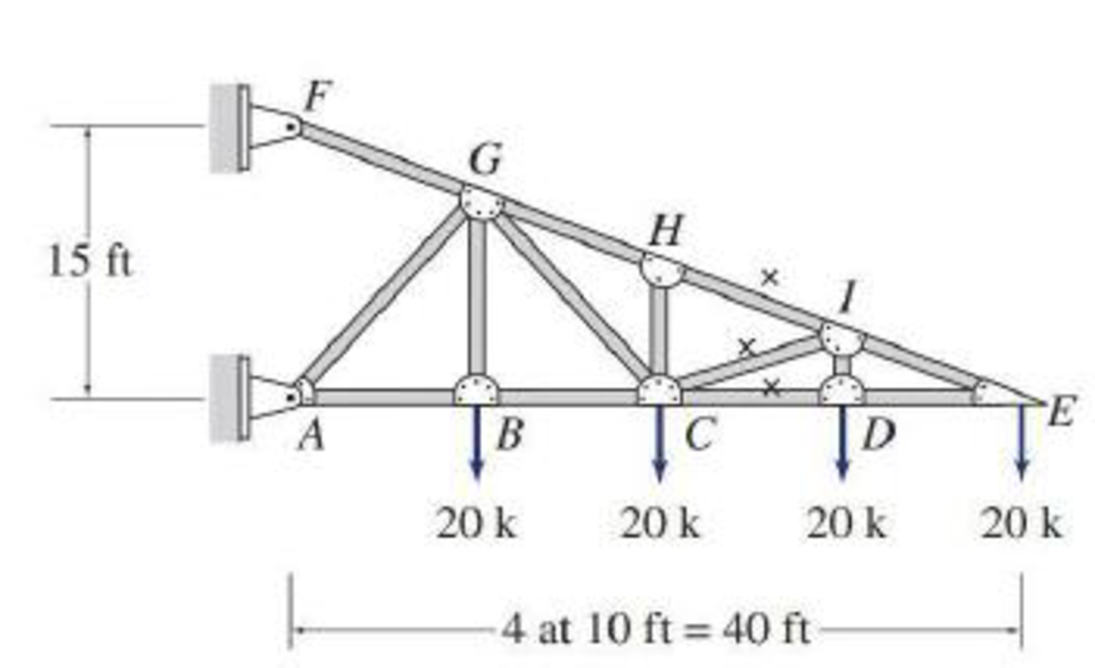# Determine the forces in the members identified by “x” of the truss shown by the method of sections. FIG. P4.38

#### Solutions

Chapter
Section
Chapter 4, Problem 38P
Textbook Problem
43 views

## Determine the forces in the members identified by “x” of the truss shown by the method of sections.FIG. P4.38

To determine

Find the forces in the members CD, CI, and HI of the truss by the method of sections.

### Explanation of Solution

Sign convention:

Apply the sign conventions for calculating reactions, forces and moments using the three equations of equilibrium as shown below.

• For summation of forces along x-direction is equal to zero (Fx=0), consider the forces acting towards right side as positive (+) and the forces acting towards left side as negative ().
• For summation of forces along y-direction is equal to zero (Fy=0), consider the upward force as positive (+) and the downward force as negative ().
• For summation of moment about a point is equal to zero (Matapoint=0), consider the clockwise moment as negative and the counter clockwise moment as positive.

Method of sections:

The negative value of force in any member indicates compression (C) and the positive value of force in any member indicates Tension (T).

The Figure of the truss is shown.

The members identified by “x” are CD, CI, and HI.

Consider the forces in the members CD, CI, and HI are denoted by FCD, FCI, and FHI.

Calculation:

Show the free body diagram of the truss as shown in Figure 1.

Refer to Figure 1.

Consider the triangles FAE and IED.

IDFA=DEAEID=DEAE×FAID=10ft40ft×15ftID=3.75ft

Consider the triangle CDI.

Consider the angle made by the member CI and IE with the horizontal as θ.

Find the angle (θ) made by the member CI and IE with the horizontal as follows:

tanθ=IDCD=IDDEθ=tan1(3.7510)θ=20.55°

Refer Figure 1

Consider a vertical section passing through the members CD, CI, and HI denoted by a-a.

Show the portion of the truss to the right of the section as shown in Figure 2.

Refer Figure 1 and Figure 2.

For Equilibrium of forces,

Take sum of moments about I as zero.

MI=0(FCD×3.75)(20×10)=0(FCD×3.75)=200FBC=(2003.75)

FBC=53.33k=53.33k(C)

Take sum of moments about E as zero

### Still sussing out bartleby?

Check out a sample textbook solution.

See a sample solution

#### The Solution to Your Study Problems

Bartleby provides explanations to thousands of textbook problems written by our experts, many with advanced degrees!

Get Started

Find more solutions based on key concepts
What is a reaction force?

Engineering Fundamentals: An Introduction to Engineering (MindTap Course List)

What e agile methods? Are they better than traditional methods? Why or why not?

Systems Analysis and Design (Shelly Cashman Series) (MindTap Course List)

Determine the resultant of the force system shown.

International Edition---engineering Mechanics: Statics, 4th Edition

What two line types work together to show sizes on an engineering drawing?

Precision Machining Technology (MindTap Course List)

What are the three primary types of public law?

Management Of Information Security

Complete Security Solutions Your neighbor started a new construction business. He would like to hire you to set...

Enhanced Discovering Computers 2017 (Shelly Cashman Series) (MindTap Course List)

Why should a memory saver be installed before dis connecting a vehicles battery?

Automotive Technology: A Systems Approach (MindTap Course List)

Why do APC ferrules create less back reflection than UPC ferrules?

Network+ Guide to Networks (MindTap Course List)

If your motherboard supports ECC DDR3 memory, can you substitute non-ECC DDR3 memory?

A+ Guide to Hardware (Standalone Book) (MindTap Course List)

What practical knowledge subject areas are covered in Module 1 of the SENSE program?

Welding: Principles and Applications (MindTap Course List)